# Prediction Models¶

A prediction model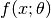is a function with two arguments: the input feature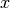and the predictor parameter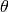. All prediction models are instances of an the abstract type PredictionModel, defined as follows:

abstract PredictionModel{NDIn, NDOut}

# NDIn:  The number of dimensions of each input (0: scalar, 1: vector, 2: matrix, ...)
# NDOut: The number of dimensions of each output (0: scalar, 1: vector, 2: matrix, ...)


## Common Methods¶

Each prediction model implements the following methods:

inputlen(pm)

Return the length of each input.

inputsize(pm)

Return the size of each input.

outputlen(pm)

Return the length of each output.

outputsize(pm)

Return the size of each output.

paramlen(pm)

Return the length of the parameter.

paramsize(pm)

Return the size of the parameter.

ninputs(pm, x)

Verify the validity of x as a single input or as a batch of inputs. If x is valid, it returns the number of inputs in array x, otherwise, it raises an error.

predict(pm, theta, x)

Predict the output given the parameter theta and the input x.

Here, x can be either a sample or an array comprised of multiple samples.

## Predefined Models¶

The package provides the following prediction models:

### (Univariate) Linear Prediction¶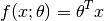• parameter:, a vector of length d.
• input::, a vector of length d.
• output:: a scalar.
immutable LinearPred <: PredictionModel{1,0}
dim::Int

LinearPred(d::Int) = new(d)
end


### (Univariate) Affine Prediction¶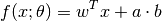Here, b is a model constant to serve as the base of the bias term.

• parameter:, a vector of length d + 1, in the form [w; a].
• input:, a vector of length d.
• output:: a scalar.
immutable AffinePred <: PredictionModel{1,0}
dim::Int
bias::Float64

AffinePred(d::Int) = new(d, 1.0)
AffinePred(d::Int, b::Real) = new(d, convert(Float64, b))
end


### (Multivariate) Linear Prediction¶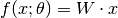• parameter: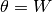, a matrix of size (k, d).
• input:, a vector of length d.
• output: a vector of length k.
immutable MvLinearPred <: PredictionModel{1,1}
dim::Int
k::Int

MvLinearPred(d::Int, k::Int) = new(d, k)
end


### (Multivariate) Affine Prediction¶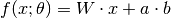Here, b is a model constant to serve as the base of the bias term.

• parameter:, a matrix of size (k, d+1), in the form [W a], where W is a coefficient matrix of size (k, d) and a is a bias-coefficient vector of size (k,).
• input:, a vector of length d.
• output: a vector of length k.
immutable MvAffinePred <: PredictionModel{1,1}
dim::Int
k::Int
bias::Float64

MvAffinePred(d::Int, k::Int) = new(d, k, 1.0)
MvAffinePred(d::Int, k::Int, b::Real) = new(d, k, convert(Float64, b))
end


## Examples¶

Here is an example that illustrates a prediction model.

pm = MvLinearPred(5, 3)   # construct a prediction model
# with input dimension 5
#      output dimension 3

inputlen(pm)     # --> 5
inputsize(pm)    # --> (5,)
outputlen(pm)    # --> 3
outputsize(pm)   # --> (3,)
paramlen(pm)     # --> 15
paramsize(pm)    # --> (3, 5)

W = randn(3, 5)     # W is a parameter matrix
x = randn(3)        # x is a single input
ninputs(pm, x)      # --> 1
predict(pm, W, x)   # make prediction: --> W * x

X = randn(3, 10)    # X is a matrix with 10 samples
ninputs(pm, X)      # --> 10
predict(pm, W, X)   # make predictions: --> W * X